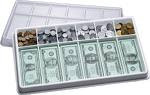google.com, pub-8985115814551729, DIRECT, f08c47fec0942fa0 Free Printable Lesson Plans: consumer math
Showing posts with label consumer math. Show all posts
Showing posts with label consumer math. Show all posts

### Free Printable Algebra, Geometry, Calculus Math Lesson Plans, Online Scientific Calculators

Algebra, geometry, calculus and trigonometry are challenging subjects for parents and students. Here are free printable algebra worksheets, trigonometry homework help, calculus tutorials and online geometry lessons to help. Links are included for free online scientific calculators and graphing calculators. Use these higher math tutorials, reference (cheat) sheets, tools and homework help online or print for home and school use.

Web Calc has free scientific calculators for homework help, including online scientific calculators. Meta-Calculator has free online graphing calculators, statistics calculators, scientific calculators and matrix calculators, unit converters and financial calculators for consumer math classes. These sites have free math tutorials as well.

Free math help has free printable math worksheets and homework help in algebra, geometry, trigonometry, statistics and calculus. Check out free printable charts, printable online tutorials, sample problems, references, explanations and study guides. Students can play online math games too. Webmath has free math worksheets, printables and tutorials on higher math like algebra, trigonometry, calculus and geometry, too. Mathway offers free online scientific calculators, online math tutorials and problem solving functions.

Purple Math provides free algebra homework help for logarithms, equalities, inequalities, formulas, equations, integers, exponents, properties, radical numbers, rational numbers, absolute value, quadratic equations, parabola, ellipse, hyperbola, circle, linear function, polynomials, factoring, square root and more. Geometry math lessons covers solids, spheres, cubes, triangles, angles, formula, Pythagorean Theorem, theorems, postulates and more. The trigonometry math lessons cover sines, cosines, tangents, Mollwied's Formula and all the various trigonometry functions and identities. Calculus math lessons cover integrals and substitutions.

### Free Printable Play Money for MathParents, if you want a sure-fire fun kids' activity, you can bank on play money being a hit. Elementary, kindergarten and preschool teachers use play currency, coins and bills in a variety of money math lessons. Here are free printable coins, bills and play money. Homeschool parents will want to use these free printable play currency for money math lessons also.

Printable Play Money has all denominations and styles of free printable coins, bills and play money you might ever need for your lessons. There are cut-out coin worksheets, counting coins, money math lessons, worksheets, activities and making change lessons. There are free printable sheets of pennies, dimes, nickels and quarters to cut out and use for counting and making change. Printable Play Money also has free printable bills and US currency in several denominations. Print play one dollar, five dollar, ten dollar, twenty dollar, fifty dollar and one hundred dollar bills. Some styles are replicas of US mint currency and some are obviously play money.